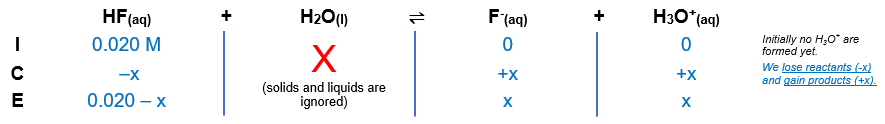# Problem: Calculate the concentration of all species present and the pH of a 0.020-M HF solution.

###### FREE Expert Solution

Equilibrium reaction:        HF(aq) + H2O ⇌ F-(aq) + H3O+

Step 1: Construct an ICE chart for the reaction.Step 2: Write the Ka expression.

${\mathbf{K}}_{{\mathbf{a}}}\mathbf{=}\frac{\mathbf{products}}{\mathbf{reactants}}\phantom{\rule{0ex}{0ex}}{\mathbf{K}}_{\mathbf{a}}\mathbf{=}\frac{\mathbf{\left[}{\mathbf{F}}^{\mathbf{-}}\mathbf{\right]}\mathbf{\left[}{\mathbf{H}}_{\mathbf{3}}{\mathbf{O}}^{\mathbf{+}}\mathbf{\right]}}{\left[\mathrm{HF}\right]}$

Ka of HF = 7.2x10-4

Step 3: Calculate the equilibrium concentrations.

${\mathbf{K}}_{\mathbf{a}}\mathbf{=}\frac{\left[\mathbf{x}\right]\left[\mathbf{x}\right]}{\left[\mathbf{0}\mathbf{.}\mathbf{020}\mathbf{-}\mathbf{x}\right]}\phantom{\rule{0ex}{0ex}}\mathbf{7}\mathbf{.}\mathbf{2}\mathbf{×}{\mathbf{10}}^{\mathbf{-}\mathbf{4}}\mathbf{=}\frac{{\mathbf{x}}^{\mathbf{2}}}{\mathbf{0}\mathbf{.}\mathbf{020}\mathbf{-}\mathbf{x}}$

null

$\mathbf{7}\mathbf{.}\mathbf{2}\mathbf{×}{\mathbf{10}}^{\mathbf{-}\mathbf{4}}\mathbf{\left(}\mathbf{0}\mathbf{.}\mathbf{020}\mathbf{-}\mathbf{x}\mathbf{\right)}\mathbf{=}\frac{{\mathbf{x}}^{\mathbf{2}}}{\overline{)\mathbf{0}\mathbf{.}\mathbf{020}\mathbf{-}\mathbf{x}}}\overline{)\left(0.020-x\right)}\phantom{\rule{0ex}{0ex}}\mathbf{1}\mathbf{.}\mathbf{44}\mathbf{×}{\mathbf{10}}^{\mathbf{-}\mathbf{5}}\mathbf{-}\mathbf{7}\mathbf{.}\mathbf{2}\mathbf{×}{\mathbf{10}}^{\mathbf{-}\mathbf{4}}\mathbf{x}\mathbf{=}{\mathbf{x}}^{\mathbf{2}}\phantom{\rule{0ex}{0ex}}\overline{)\mathbf{0}\mathbf{=}{\mathbf{x}}^{\mathbf{2}}\mathbf{+}\mathbf{7}\mathbf{.}\mathbf{2}\mathbf{×}{\mathbf{10}}^{\mathbf{-}\mathbf{4}}\mathbf{-}\mathbf{1}\mathbf{.}\mathbf{44}\mathbf{×}{\mathbf{10}}^{\mathbf{-}\mathbf{5}}}$

Now, we will use the quadratic formula to solve for x. The quadratic formula is:

$\overline{){\mathbf{x}}{\mathbf{=}}\frac{\mathbf{-}\mathbf{b}\mathbf{±}\sqrt{{\mathbf{b}}^{\mathbf{2}}\mathbf{-}\mathbf{4}\mathbf{a}\mathbf{c}}}{\mathbf{2}\mathbf{a}}}$

$\mathbf{x}\mathbf{=}\frac{\mathbf{-}\mathbf{7}\mathbf{.}\mathbf{2}\mathbf{×}{\mathbf{10}}^{\mathbf{-}\mathbf{4}}\mathbf{±}\sqrt{\mathbf{\left(}\mathbf{7}\mathbf{.}\mathbf{2}\mathbf{×}{\mathbf{10}}^{\mathbf{-}\mathbf{4}}{\mathbf{\right)}}^{\mathbf{2}}\mathbf{-}\mathbf{4}\mathbf{\left(}\mathbf{1}\mathbf{\right)}\mathbf{\left(}\mathbf{-}\mathbf{1}\mathbf{.}\mathbf{44}\mathbf{×}{\mathbf{10}}^{\mathbf{-}\mathbf{5}}\mathbf{\right)}}}{\mathbf{2}\mathbf{\left(}\mathbf{1}\mathbf{\right)}}\phantom{\rule{0ex}{0ex}}\mathbf{x}\mathbf{=}\frac{\mathbf{-}\mathbf{7}\mathbf{.}\mathbf{2}\mathbf{×}{\mathbf{10}}^{\mathbf{-}\mathbf{4}}\mathbf{±}\sqrt{\mathbf{5}\mathbf{.}\mathbf{184}\mathbf{×}{\mathbf{10}}^{\mathbf{-}\mathbf{7}}\mathbf{+}\mathbf{5}\mathbf{.}\mathbf{76}\mathbf{×}{\mathbf{10}}^{\mathbf{-}\mathbf{5}}}}{\mathbf{2}}\phantom{\rule{0ex}{0ex}}\mathbf{x}\mathbf{=}\frac{\mathbf{-}\mathbf{7}\mathbf{.}\mathbf{2}\mathbf{×}{\mathbf{10}}^{\mathbf{-}\mathbf{4}}\mathbf{±}\sqrt{\mathbf{5}\mathbf{.}\mathbf{81184}\mathbf{×}{\mathbf{10}}^{\mathbf{-}\mathbf{5}}}}{\mathbf{2}}\phantom{\rule{0ex}{0ex}}\mathbf{x}\mathbf{=}\frac{\mathbf{-}\mathbf{7}\mathbf{.}\mathbf{2}\mathbf{×}{\mathbf{10}}^{\mathbf{-}\mathbf{4}}\mathbf{±}\mathbf{7}\mathbf{.}\mathbf{62}\mathbf{×}{\mathbf{10}}^{\mathbf{-}\mathbf{3}}}{\mathbf{2}}$

The calculation then splits into two ways:

92% (373 ratings)###### Problem Details

Calculate the concentration of all species present and the pH of a 0.020-M HF solution.

Frequently Asked Questions

What scientific concept do you need to know in order to solve this problem?

Our tutors have indicated that to solve this problem you will need to apply the Weak Acids concept. If you need more Weak Acids practice, you can also practice Weak Acids practice problems.

What professor is this problem relevant for?

Based on our data, we think this problem is relevant for Professor Halpin's class at NYU.

What textbook is this problem found in?

Our data indicates that this problem or a close variation was asked in Chemistry: An Atoms First Approach - Zumdahl Atoms 1st 2nd Edition. You can also practice Chemistry: An Atoms First Approach - Zumdahl Atoms 1st 2nd Edition practice problems.